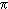# FAQ: Probability that gcd(n,m) = 1?

### By Chris Caldwell

Here is a frequently asked question

While playing with programs to determine primes and relative primes, I stumbled over an interesting (at least to me) fact. While the probability of a random number being prime decreases as the range of possible random numbers increases (Prime Number Theorem), the probability of two random numbers being relatively prime is 60.8% Is this something that is either well known by or trivially obvious to prime number gurus?

That there should be such a constant is "obvious", finding its value takes more work. In 1849 Dirichlet showed that the probability is exactly 6/π2 arguing roughly as follows.

Suppose you pick two random numbers less than n, then

• [n/2]2 pairs are both divisible by 2.
• [n/3]2 pairs are both divisible by 3.
• [n/5]2 pairs are both divisible by 5.
• ...

(Here [x] is the greatest integer less than or equal to x, usually called the floor function.) So the number of relatively prime pairs less than or equal to n is (by the inclusion/exclusion principle):

n2 - Σ([n/p]2) + Σ([n/pq]2) - Σ([n/pqr]2) + ...

where the sums are taken over the primes p, q, r,... less than n. Letting μ(x) be the möbius function this is

Σ(μ(k)[n/k]2)     (sum over positive integers k
so the desired constant is the limit as n goes to infinity of this sum divided by n2, or
Σ(μ(k)/k2)     (sum over positive integers k).
But this series times the sum of the reciprocals of the squares is one, so the sum of this series, the desired limit, is 6/2. This number is approximately
60.7927101854026628663276779258365833426152648033479
percent.  ;-)